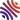## 25 publications1. Topology from enrichment: the curious case of partial metrics ( and ), In Cah. Topol. G'eom. Diff'er. Cat'eg., volume 59, . [hal-lmpa]
2. The double power monad is the composite power monad (), In Fuzzy Sets and Systems, volume 313, . [hal-lmpa]
3. An introduction to quantaloid-enriched categories (), In Fuzzy Sets and Systems, volume 256, . [hal-lmpa]
4. Grothendieck quantaloids for allegories of enriched categories ( and ), In Bull. Belg. Math. Soc. Simon Stevin, volume 19, . [hal-lmpa]
5. Elementary characterisation of small quantaloids of closed cribles ( and ), In J. Pure Appl. Algebra, volume 216, . [hal-lmpa]
6. Symmetry and Cauchy completion of quantaloid-enriched categories ( and ), In Theory Appl. Categ., volume 25, . [hal-lmpa]
7. Towards Stone duality for topological theories ( and ), In Topology Appl., volume 158, . [hal]
8. "Hausdorff distance" via conical cocompletion (), In Cah. Topol. Géom. Différ. Catég., volume 51, . [hal-lmpa]
9. On principally generated quantaloid-modules in general, and skew local homeomorphisms in particular ( and ), In Ann. Pure Appl. Logic, volume 161, . [hal]
10. Modules on involutive quantales: canonical Hilbert structure, applications to sheaf theory ( and ), In Order, volume 26, . [hal]
11. Exponentiable functors between quantaloid-enriched categories (, and ), In Appl. Categ. Structures, volume 17, . [hal]
12. $\scr Q$-modules are $\scr Q$-suplattices (), In Theory Appl. Categ., volume 19, . [hal]
13. Towards "dynamic domains": totally continuous cocomplete $\scr Q$-categories (), In Theoret. Comput. Sci., volume 373, . [hal]
14. Propositional systems, Hilbert lattices and generalized Hilbert spaces ( and ), Chapter in Handbook of quantum logic and quantum structures, Elsevier Sci. B. V., Amsterdam, . [hal]
15. Categorical structures enriched in a quantaloid: tensored and cotensored categories (), In Theory Appl. Categ., volume 16, . [hal]
16. The canonical topology on a meet-semilattice (), In Internat. J. Theoret. Phys., volume 44, .
17. Categorical structures enriched in a quantaloid: orders and ideals over a base quantaloid (), In Appl. Categ. Structures, volume 13, . [hal]
18. Categorical structures enriched in a quantaloid: regular presheaves, regular semicategories (), In Cah. Topol. Géom. Différ. Catég., volume 46, . [hal]
19. Categorical structures enriched in a quantaloid: categories, distributors and functors (), In Theory Appl. Categ., volume 14, . [hal]
20. Quantaloids describing causation and propagation of physical properties (, and ), In Found. Phys. Lett., volume 14, . [hal]
21. Short introduction to enriched categories ( and ), Chapter in Current research in operational quantum logic, Kluwer Acad. Publ., Dordrecht, volume 111, . [hal]
22. State transitions as morphisms for complete lattices ( and ), Chapter in , volume 39, . [hal]
23. On a duality of quantales emerging from an operational resolution ( and ), Chapter in , volume 38, .
24. Operational resolutions and state transitions in a categorical setting ( and ), In Found. Phys. Lett., volume 12, . [hal]
25. How quantales emerge by introducing induction within the operational approach (, and ), In Helv. Phys. Acta, volume 71, . [hal]